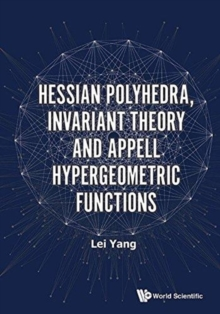### Next Day Delivery Available# Hessian Polyhedra, Invariant Theory And Appell Hypergeometric Functions Hardback

#### Description

Our book gives the complex counterpart of Klein's classic book on the icosahedron.

We show that the following four apparently disjoint theories: the symmetries of the Hessian polyhedra (geometry), the resolution of some system of algebraic equations (algebra), the system of partial differential equations of Appell hypergeometric functions (analysis) and the modular equation of Picard modular functions (arithmetic) are in fact dominated by the structure of a single object, the Hessian group \$mathfrak{G}'_{216}\$.

It provides another beautiful example on the fundamental unity of mathematics.

#### Information

• Format: Hardback
• Pages: 316 pages
• Publisher: World Scientific Publishing Co Pte Ltd
• Publication Date:
• Category: Calculus & mathematical analysis
• ISBN: 9789813209473

£123.00

£94.71